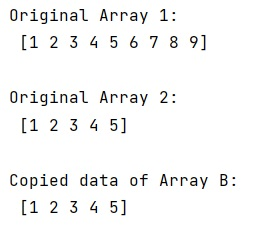# How to copy data from a NumPy array to another?

Learn, how to copy data from a NumPy array to another in Python?
Submitted by Pranit Sharma, on January 10, 2023

NumPy is an abbreviated form of Numerical Python. It is used for different types of scientific operations in python. Numpy is a vast library in python which is used for almost every kind of scientific or mathematical operation. It is itself an array which is a collection of various methods and functions for processing the arrays.

## Copying data from a NumPy array to another

Suppose that we are given two NumPy arrays and we need to find the fastest way to copy the data of this array into another array without modifying the address of the array.

For this purpose, we will first use numpy.empty_like() method which returns a new array with the same shape and type as a given array. Finally, we will copy all the data of B into original array.

Let us understand with the help of an example,

## Python code to copy data from a NumPy array to another

```# Import numpy
import numpy as np

# Creating two arrays
arr = np.array([1,2,3,4,5,6,7,8,9])
B = np.array([1,2,3,4,5])

# Display original arrays
print("Original Array 1:\n",arr,"\n")
print("Original Array 2:\n",B,"\n")

# Using empty_like method
arr = np.empty_like(B)

# Copying data of B into arr
arr[:] = B

# Display result
print("Copied data of Array B:\n",arr,"\n")
```

Output: